Introduction to coveffectsplot

2020-12-10

The use of forest plots to summarize the impact of various intrinsic and extrinsic factors on the pharmacokinetics (PK) of drugs is becoming a standard practice and a key part of submission packages to the FDA. The forest plots format make it easier for clinicians to quickly find and interpret the information they need.1

Motivation

Traditionally, a paragraph describing the various doses in various group is part of the official drug label and often a table is provided. The advantages of table versus graphs has been previously discussed and each has its own merits. While web-based interactive graphics allow on-demand mouse hovering to show the graph numbers, this is not possible on a printed sheet of paper or on a static PDF as required for a drug label. As such, combining a graphic representation of the data with a side table provides the best of both worlds and provide to the clinician an efficient way to interpret the data.

Background on a Fictitious Drug

Let us assume that we have a drug following a first-order absorption one-compartment PK model with parameters absorption constant (Ka), Clearance (CL) and Volume of distribution (V). Usually, a nonlinear mixed effects model is fitted to the PK data and covariates covering intrinsic and extrinsic are tested on the various parameters. For simplicity, let us assume that the covariate modeling did not add any covariate on Ka and V and provided the following model for CL:

$CL = {POPCL} \times \left( \frac { \color{blue}{Weight}} {70}\right)^{dWTdCL}\times \left( dSexdCL\times \left( \color{blue}{Sex}== 1 \right) \right)\times \left( exp(\eta{CL})\right)$

The above equation shows that we have two covariates on CL one is Weight (kg) a continuous variable with reference value of 70 (kg) and influencing CL with a power model with coefficient dWTdCL. The second is Sex which is an indicator variable taking the value of 0 (Woman, used as the reference category) and 1 (Man) influencing CL with a coefficient dSexdCL. The last term denotes the individual deviations from the population (random effects) which assumes that CL in the population is log normally distributed. The same model can be more familiar to statisticians if re-written into a log-linear additive form:

$log(CL) = {log(POPCL)} + dWTdCL\times log\left(\frac { \color{blue}{Weight}} {70}\right)+ \ log(dSexdCL)\times\left(\color{blue}{Sex}== 1 \right) +\eta{CL}$ and where the individual level random effect describes the between subject variability (BSV) and is: $\eta{CL}\sim \mathcal{N}(0,\,\omega_{CL}^{2})$ The modeling output would give you the value of the fixed effects parameters (POPCL, dWTdCL and dSexdCL), the variance covariance matrix of the random effects as well as the associated uncertainty from the estimated asymptotic variance covariance matrix of the various estimated parameters. Alternatively, the uncertainty can also be obtained using a nonparametric bootstrap resampling of the individuals. Oftentimes, the uncertainty is reported as a standard error or relative standard error (%). If we are interested in reporting the standard error of CL in Men there is several ways we can use to compute it: 1) error propagation using the delta method. 2) simulation from the variance covariance matrix. 3) using the bootstrap distribution.

The observed distribution of the covariates Weight and Sex in the studied population is important because, to compute the covariates effects, we need to choose values for all covariates included in the model. It is desirable to provide sensible values that would provide a good sense on where most of the patients are. A common practice is to report the effects of the 75th percentile to the 25th percentile which will cover 50% of the population, some would also want to cover 90% of the population (5th to the 95th percentiles). Of note is that we need to cover enough of the covariate range and steps(e.g. show effects at the 5th, 25th, 50th, 75th and 95th percentiles) to illustrate a nonlinear relationship like the power model.

Alternatively, we might be interested to compute effects for clinically meaningful difference e.g. 20 kg and then we report effects at 50, 70 and 90 kg. Some clinical covariates like eGFR have predefined cutoffs that we want to cover.

If the assumption of varying one covariate at a time does not hold we recommend the use of a realistic distribution of correlated covariates (simulated or real).

Finally, since the BSV cannot be controlled for, showing the distribution of the BSV is important to contrast and compare with the estimated covariate related effects as this will allow us to understand where a random subject given a known set of covariates could possibly belong. We also suggest that precomputing, BSV ranges based on percentiles of the BSV distribution is more helpful than letting the user guess the ranges from visual inspection.

Simulating Data and Outputs from a Modeling Exercise

We will assume that the model fit had estimated parameters with relative standard errors of 15%. For this vignette, a simulation from a multivariate normal distribution with n= 10000 was used. The five first rows are shown in the table below. The assumed mean values for POPCL, dWTdCL and dSexdCL were 10, 0.75 and 1.5 respectively. For simplicity, we will also assume that there were equal number of Sex = 1 (Man) and Sex = 0 (Woman) and that men and women had mean weights of 75 and 65 kg and the same standard deviation of 20 kg. Note that unless we explicitly code the simulation in a way to prevent negative weights (e.g. using a log normal distribution) we will end up simulating some negative ones.

set.seed(657687)
df <- data.frame(
MASS::mvrnorm(n = nuncertainty,
mu = c(10,0.75,1.5),
Sigma=matrix(c((10*0.15)^2,
0.001,0.001,0.001,(0.75*0.15)^2,
0.001,0.001,0.001,(1.5*0.15)^2),3,3,byrow = TRUE)
))
names(df) <- c("POPCL","dWTdCL","dSexdCL")
POPCL dWTdCL dSexdCL
9.14 0.83 1.20
10.65 0.82 1.51
12.30 0.88 1.56
9.40 0.88 1.81
10.37 0.85 1.51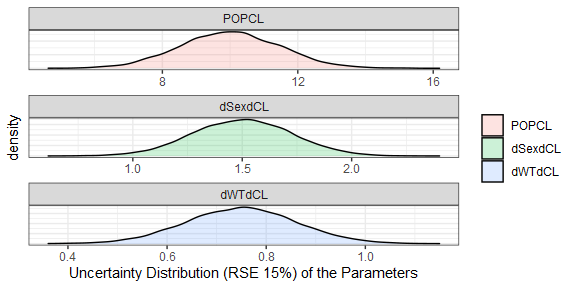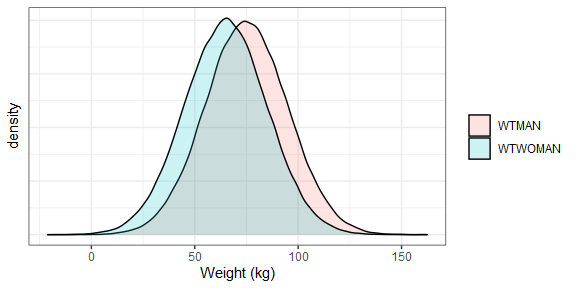1% 5% 25% 50% 75% 95% 99%
22 36 56 70 84 104 118

The model had a between subject variability on CL $$\omega_{CL}^{2}$$ variance of 0.09 which translates to apparent CV of sqrt (exp (0.09) -1) = 0.3069. A common way to report this BSV is to say we have 30.7% BSV. But what does this really mean in practical terms? What are the chances that a patient with known covariate values, will have very low or very high CL warranting dose changes?
A useful metric can be to compute the bounds where say 50% and 90% of the patients will be located using simple quantile functions on simulated distributions. For the 30.7% BSV case, we compute that 50% of the patients will be within the 0.82 to 1.23 interval (dark red area) while 90% of the patients will be within the 0.61 to 1.63 interval (lighter red area). A table showing the various quantiles is also shown. For asymmetrical distribution we can also use the highest density intervals instead of percentiles but this is not shown here.

set.seed(546789)
CLBSVdistribution <- data.frame(CL= 10*exp(rnorm(nbsvsubjects,0,sd=0.09^0.5)))
CLBSVdistribution$CLBSV<- CLBSVdistribution$CL/10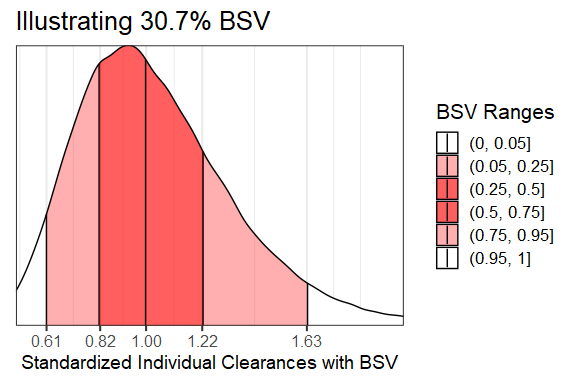1% 5% 25% 50% 75% 95% 99%
0.49 0.61 0.82 1.00 1.22 1.63 2.01

Visualizing Covariate Effects with Distributions

• First, we will divide the POPCL uncertainty distribution by its median to standardize it. This will make everything relative to the reference where all covariates are held at the reference value(s) here Sex = 0 and Weight = 70 kg.
• Second, We will compute the effects of Weight = 50 kg and 90 kg as compared to the 70 kg. We keep dSexdCL as is, it represents the effects of Sex = 1 effects when weight is held at its reference value = 70 kg.
• Third, we optionally compute the effects for combination of covariates of interest e.g. Weight = 90 kg and Sex = 1. A clinical relevance areas e.g. between 0.8 and 1.25 of the reference value is shown since this is often regarded as the zone of PK equivalence. Usually, a covariate need to have effects driving PK exposures outside of this zone to trigger actions requiring dose changes. * Finally, we will make a plot that compare the magnitude of uncertainty, covariate distribution and between subject variability effects.
dfeffects <- df
dfeffects$REF <- dfeffects$POPCL/ median(dfeffects$POPCL) dfeffects$SEX_FEMALE_WT_50 <- dfeffects$REF*(50/70)^dfeffects$dWTdCL
dfeffects$SEX_FEMALE_WT_90 <- dfeffects$REF*(90/70)^dfeffects$dWTdCL dfeffects$SEX_Male_WT_70 <- dfeffects$dSexdCL dfeffects$SEX_Male_WT_90 <- dfeffects$dSexdCL*dfeffects$REF*(90/70)^dfeffects$dWTdCL dfeffects$BSV<-  sample(CLBSVdistribution$CLBSV, nuncertainty) dfeffects<- dfeffects[,c("SEX_FEMALE_WT_50", "SEX_FEMALE_WT_90", "SEX_Male_WT_70", "SEX_Male_WT_90", "REF", "BSV")] dflong <- tidyr::gather(dfeffects) ggplot2::ggplot(dflong,ggplot2::aes(x=value,y=key,fill=factor(..quantile..)))+ ggridges::stat_density_ridges( geom = "density_ridges_gradient", calc_ecdf = TRUE, quantile_lines = TRUE, rel_min_height = 0.01, quantiles = c(0.05,0.5, 0.95)) + ggplot2::scale_fill_manual( name = "Probability", values = c("#FF0000A0", "white","white", "#0000FFA0"), labels = c("(0, 0.05]", "(0.05, 0.5]","(0.5, 0.95]", "(0.95, 1]") )+ ggplot2::annotate( "rect", xmin = 0.8, xmax = 1.25, ymin = -Inf, ymax = Inf, fill = "gray",alpha=0.4 )+ ggplot2::geom_vline( ggplot2::aes(xintercept = 1), size = 1 )+ ggplot2::theme_bw()+ ggplot2::labs(x="Effects Relative to parameter reference value",y="")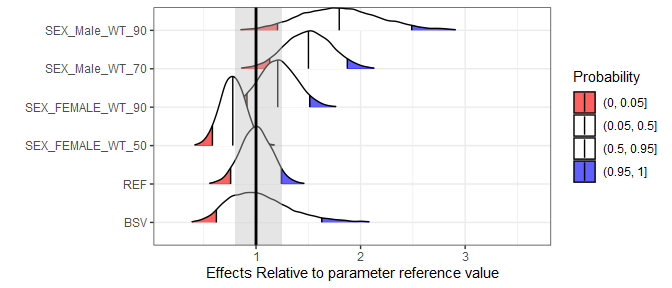Here we overlay the various sources of variability to compare them head to head: ggplot2::ggplot(dfeffects)+ ggplot2::geom_density(ggplot2::aes(x=REF,y=..scaled..,col="a.Uncertainty\nRSE=15%"))+ ggplot2::geom_density(data=dfcovlong, ggplot2::aes(x=(value/70)^0.75 , y=..scaled..,col="b.Weight\nMean=70 kg, sd=20 kg"))+ ggplot2::geom_density(data=CLBSVdistribution ,ggplot2::aes(x=CLBSV, y=..scaled..,col="c.Between subject variability\nCV=30%"))+ ggplot2::theme_bw(base_size = 16)+ ggplot2::theme(axis.text.y = ggplot2::element_blank(), axis.ticks.y = ggplot2::element_blank())+ ggplot2::scale_x_continuous(breaks=c(0.25,0.5,0.8,1,1.25,1.5,2,3))+ ggplot2::coord_cartesian(xlim=c(0.25,2))+ ggplot2::labs(color="",x="Effects Standardized Relative to the Typical Value",y= "Scaled Density")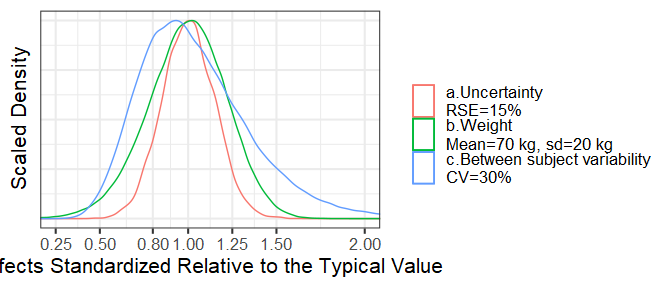Simplifying the Distributions into Ranges of Effects The above plots might be overloading the reader with information. We will simplify it by removing unnecessary details and by computing the desired stats in advance. dfeffects$SEX_Male_WT_90<- NULL
dfeffectslong<- tidyr::gather(dfeffects)
dfeffectslong<- dplyr::group_by(dfeffectslong,key)
dfeffectslongsummaries<- dplyr::summarise(dfeffectslong,mid=quantile(value,0.5),
lower=quantile(value,0.05),
upper=quantile(value,0.95))

dfeffectslongsummaries$paramname <- "CL" dfeffectslongsummaries$covname <- c("BSV","REF","Weight","Weight","Sex")
dfeffectslongsummaries$label <- c("95% of patients","70 kg/Woman", "50 kg/Woman", "90 kg/Woman","70 kg/Man") dfeffectslongsummaries<- rbind(dfeffectslongsummaries, data.frame(key=c("BSV","BSV"), mid=c(quantile(dfeffects$BSV,0.5), quantile(dfeffects$BSV,0.5)), lower = c(quantile(dfeffects$BSV,0.25), quantile(dfeffects$BSV,0.05)), upper = c(quantile(dfeffects$BSV,0.75), quantile(dfeffects$BSV,0.95)), paramname= "CL", covname=c("BSV","BSV"), label = c("50% of patients","90% of patients") ) ) dfeffectslongsummaries<- dfeffectslongsummaries[c(2,6,7,3,4,5),] plotdata <- dplyr::mutate(dfeffectslongsummaries, LABEL = paste0(format(round(mid,2), nsmall = 2), " [", format(round(lower,2), nsmall = 2), "-", format(round(upper,2), nsmall = 2), "]")) plotdata<- as.data.frame(plotdata) plotdata<- plotdata[,c("paramname","covname","label","mid","lower","upper","LABEL")] knitr::kable(plotdata) paramname covname label mid lower upper LABEL CL REF 70 kg/Woman 1.0000000 0.7571492 1.2419051 1.00 [0.76-1.24] CL BSV 50% of patients 1.0032493 0.8173299 1.2180588 1.00 [0.82-1.22] CL BSV 90% of patients 1.0032493 0.6209209 1.6280838 1.00 [0.62-1.63] CL Weight 50 kg/Woman 0.7776982 0.5830118 0.9753821 0.78 [0.58-0.98] CL Weight 90 kg/Woman 1.2074765 0.9136419 1.5132128 1.21 [0.91-1.51] CL Sex 70 kg/Man 1.5012342 1.1307812 1.8728976 1.50 [1.13-1.87] Plotting the Effects Data First we do a customized ggplot but we quickly notice that it has some issues like the lack of legend for the clinical reference area, vertical labels etc. We then show how using coveffectsplot::forest_plot can generate a plot annotations, a side table with values, and legends. For interactive reordering of categories, editing of labels and more, export the data as a “csv” and launch the shiny app via coveffectsplot::run_interactiveforestplot(). plotdata$covname <- as.factor(plotdata$covname) plotdata$covname <- reorder(plotdata$covname , c(3,4,4,2,1,1)) plotdata$label <- reorder(as.factor(plotdata\$label) , c(1,3,2,4,5,6))
ggplot2::ggplot(data = plotdata, ggplot2::aes_string(
y = "label",
x = "mid",
xmin = "lower",
xmax = "upper"
)) +
ggstance::geom_pointrangeh(
position = ggstance::position_dodgev(height = 0.75),
ggplot2::aes(color = "90 %CI\nCovariate Effects"),
size = 1,
alpha = 1
)+
ggplot2::annotate("rect", xmin = min(0.8),
xmax = max(1.25), ymin = -Inf, ymax = Inf, fill = "gray",alpha=0.1)+
ggplot2::geom_vline(ggplot2::aes(xintercept = 1,linetype="Reference"))+
ggplot2::facet_grid(covname~.,scales="free_y",switch="y")+
ggplot2::labs(y="",x="Effects Relative to Reference Value",
colour="",linetype="")+
ggplot2::theme_bw()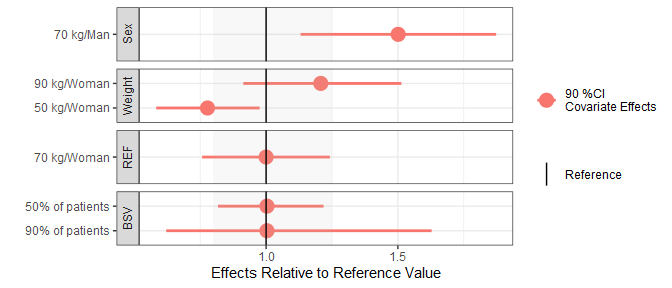png("./coveffectsplot.png",width =9 ,height = 6,units = "in",res=72)
coveffectsplot::forest_plot(plotdata,
ref_area = c(0.8, 1/0.8),
x_facet_text_size = 13,
y_facet_text_size = 13,
interval_legend_text = "Median (points)\n90% CI (horizontal lines)",
ref_legend_text = "Reference (vertical line)\n+/- 20% ratios (gray area)",
area_legend_text = "Reference (vertical line)\n+/- 20% ratios (gray area)",
xlabel = "Fold Change Relative to Parameter",
facet_formula = "covname~.",
facet_switch = "both",
facet_scales = "free",
facet_space = "fixed",
paramname_shape = TRUE,
show_table_facet_strip = "none",
table_position = "right",
table_text_size=4,
plot_table_ratio = 4,
legend_space_x_mult = 0.5,
return_list = FALSE)
dev.off()
#> png
#>   2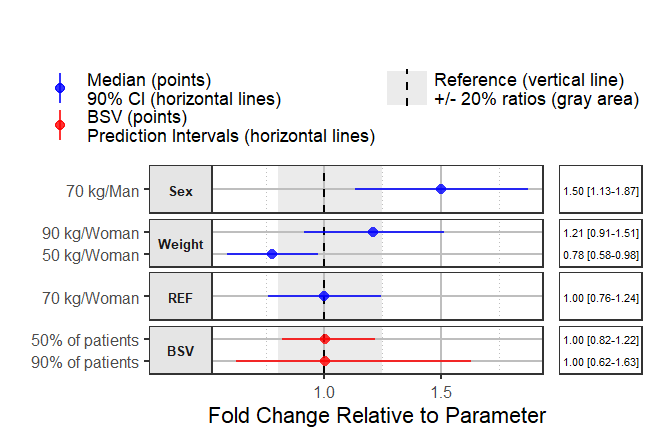Using interactive graphics with hover on-demand functionality would remove the need for a side table, this can be achieved using plotly. The code is included but not evaluated to keep the size of the vignette small.

plotdata<- plotdata[ c(3,2,1,4,5,6),]
plotly::plot_ly(plotdata) %>%
x = ~ round(lower, 2),
xend = ~ round(upper, 2),
y = ~ label,
yend = ~ label,
name = '90%CI',
line = list(color = plotly::toRGB("blue", alpha = 0.5), width = 5),
hoverinfo = "text",
text = ~ paste("</br> 90%CI: ",
paste(round(lower, 2), round(upper, 2)))
) %>%
x = ~ round(mid, 2),
y = ~ label,
name = "Median",
marker  = list(
color = plotly::toRGB("black", alpha = 0.3),
size = 20,
symbol = "diamond"
),
hoverinfo = "text",
text = ~ paste("</br> Median: ",
paste(round(mid, 2)))
) %>%
plotly::layout(
xaxis = list(
title = 'Effects Relative to Reference',
ticks = "outside",
autotick = TRUE,
ticklen = 5,
gridcolor = plotly::toRGB("gray50"),
showline = TRUE
) ,
yaxis = list (
title = '' ,
autorange = TRUE,
type = "category",
categoryorder = "trace",
ticks = "outside",
autotick = TRUE,
ticklen = 5,
gridcolor = plotly::toRGB("gray50"),
showline = TRUE
),
shapes =list(
type = "rect",
x0 = 0.8,
x1 = 1.25,
xref = "x",
yref = "paper",
y0 = 0,
y1 = 1,
line = list(width = 0),
fillcolor =  plotly::toRGB("black", alpha = 0.2)
)
)

The return_list option allows you to choose to return a list of ggplot objects that can be further manipulated.

png("./coveffectsplot2.png",width =9 ,height = 6,units = "in",res=72)
plotlist<- coveffectsplot::forest_plot(plotdata,
ref_area = c(0.8, 1/0.8),
x_facet_text_size = 13,
y_facet_text_size = 13,
interval_legend_text = "Median (points)\n90% CI (horizontal lines)",
ref_legend_text = "Reference\n(vertical line)\n+/- 20% ratios\n(gray area)",
area_legend_text = "Reference\n(vertical line)\n+/- 20% ratios\n(gray area)",
xlabel = "Fold Change Relative to Parameter",
facet_formula = "covname~.",
facet_switch = "both",
facet_scales = "free",
facet_space = "fixed",
paramname_shape = FALSE,
table_position = "right",
table_text_size = 4,
plot_table_ratio = 4,
show_table_facet_strip = "none",
legend_space_x_mult = 0.5,
ref_area_col = rgb( col2rgb("gray50"), col2rgb("gray50"),col2rgb("gray50"),
max = 255, alpha = 0.1*255 ) ,
interval_col = "steelblue",
return_list = TRUE)
egg::ggarrange(
plotlist[]+
ggplot2::labs(x= expression(paste("Changes Relative to ",
CL["subscript"]^alpha["beta"], " Reference"),
sep=""))+
ggplot2::theme(strip.text.y =  ggplot2::element_text(colour="blue")),
plotlist[] ,
nrow = 1,
widths = c(4, 1)
)

dev.off()
#> png
#>   2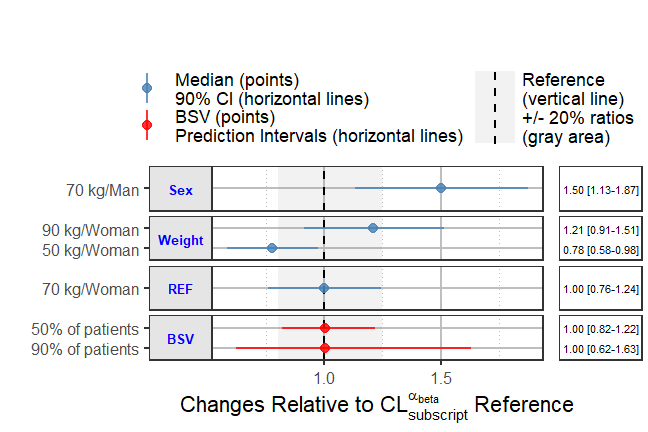In this introductory vignette we covered univariate covariate effects where we vary one at a time. Refer to the other vignettes for more advanced examples illustrating full simulation of PK or PK/PD or exposure-response models including the use of full covariate distributions.

1. Essential pharmacokinetic information for drug dosage decisions: a concise visual presentation in the drug label. Clin Pharmacol Ther. 2011 Sep;90(3):471-4.↩︎